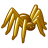PSoC™ 5, 3 & 1Level 1
Level 1

# [TrueTouch] Data rate from Touch controller to Host µC?

Hello,

we are trying to estimate the required data rate / bandwidth from the touch controller (CYAT8168) to the host µC.

The max. refresh rate of the CYAT8168 is 250 Hz. I assume we get some bytes (lets assume 4) for the x/y position of each touch event.

So, I guess the max. data rate is something like

250 Hz * 4 Byte = 1000Byte/s

Is this estimation approximately correct?

0 Replies